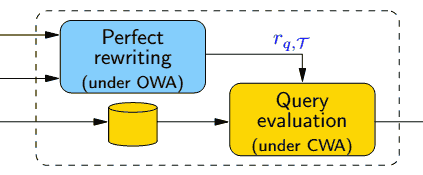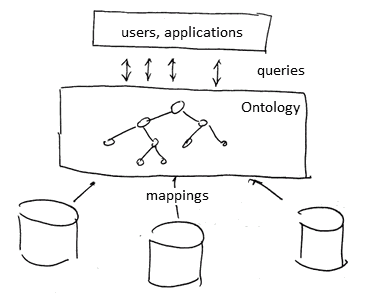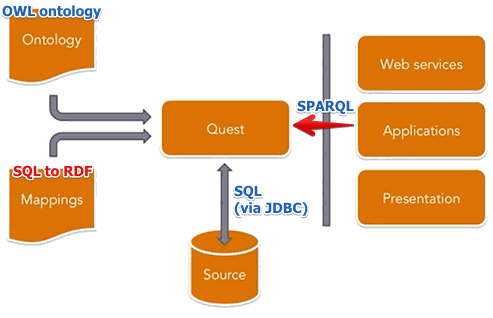# ML Wiki

## Ontology Based Data Access

For querying ontologies

• typically using SPARQL
• keep data in a db (or a triple store), but access it via ontologies
• as a bonus, have inference capabilities during query answering, since it's based on Logic
• also useful for Data Integration

• In DBMS all facts are explicit, but in Semantic Web, there are inferred tuples
• Constraints: can't violate in RDBMs, additional facts are inferred in SW to satisfy the constraints

Use SPARQL for querying ontologies

Example:

SELECT ?x WHERE {
?x :EnrolledIn ?y .
?z rdf:type :Professor .
}


Translation:

• FOL: $Q(x) \equiv \forall x \ \exists \ y, z \ : \ \text{EnrolledIn}(x, y) \land \text{Leads}(z, y) \land \text{Professor}(z)$
• CQ: $Q(x) \leftarrow \text{EnrolledIn}(x, y), \text{Leads}(z, y), \text{Professor}(z)$

### Inference Approaches

But since the ontologies are backed by some storage,

• need to make sure that inference happens
• otherwise we will just query facts not backed by TBox of our ontology

#### Main Approaches

Cached Inference

• inferred triples are stored along with asserted
• risk an explosion of the triple store
• also, change management is important - how to propagate changes and deletes
• for deletes - same inferred tuple can be due to several facts, so need to be careful when deleting

Just-In-Time Inference

• To respond to queries only
• no inferred triples retained

Compromise

• can be materialization of some inferences tuples

### Just-In-Time Inference

Query

• (for ABox and TBox, see Descriptive Logic)
• using both ABox (facts - RDF graph) and TBox (rules - Ontology)
• a triple is in an answer set either
• because it's in the ABox
• or it's a consequence of some fact from ABox inferred by the TBox

Note:

• consists of two phases
• query reformulation (rewriting)
• translate the original query $q$ into a set of queries $Q$
• reasoning happens here: Only TBox is accessed
• algorithm for rewriting: #Perfect Rewriting
• query execution
• for each $q_i \in \{ q \} \cap Q$
• execute $q_i$ against the ABox
• (simply evaluating $q$ will give us an incomplete result)#### Example

Consider this query $Q(x)$:

• $Q(x) \leftarrow \text{EnrolledIn}(x, y), \text{Leads}(z, y), \text{Professor}(z)$ can become:

Set of rules (FOL notation)

• $\text{AcademicStaff} (X) \Rightarrow \text{Staff} (X)$
• $\text{Professor}(X) \Rightarrow \text{AcademicStaff} (X)$
• $\text{Lecturer}(X) \Rightarrow \text{AcademicStaff} (X)$
• $\text{PhDStudent}(X) \Rightarrow \text{Lecturer}(X)$
• $\text{PhDStudent}(X) \Rightarrow \text{Student}(X)$
• $\text{TeachesIn}(X, Y) \Rightarrow \text{AcademicStaff}(X)$
• $\text{TeachesIn}(X, Y) \Rightarrow \text{Course}(Y)$
• $\text{ResponsibleOf} (X, Y) \Rightarrow \text{Professor}(X)$
• $\text{ResponsibleOf} (X, Y) \Rightarrow \text{Course}(Y)$
• $\text{TeachesTo}(X, Y) \Rightarrow \text{AcademicStaff} (X)$
• $\text{TeachesTo}(X, Y) \Rightarrow \text{Student}(Y)$
• $\text{Leads}(X, Y) \Rightarrow \text{AdministrativeStaff} (X)$
• $\text{Leads}(X, Y) \Rightarrow \text{Dept}(Y)$
• $\text{RegisteredIn}(X, Y) \Rightarrow \text{Student}(X)$
• $\text{RegisteredIn}(X, Y) \Rightarrow \text{Course}(Y)$
• $\text{ResponsibleOf}(X, Y) \Rightarrow \text{TeachesIn}(X, Y)$
• $\text{Professor}(X) \Rightarrow \exists Y \ : \ \text{TeachesIn}(X, Y)$
• $\text{Course}(X) \Rightarrow \exists Y \ : \ \text{RegisteredIn}(Y, X)$
• $\text{Student}(X) \Rightarrow \lnot \text{Staff} (X)$

The following are reformulations of $Q(x)$

• $q_{1}(x) \leftarrow \text{ResponsibleOf}(x,y), \text{RegisteredIn}(z,y), \text{Student}(z)$
• $q_{2}(x) \leftarrow \text{TeachesIn}(x,y), \text{RegisteredIn}(z,y), \text{PhDStudent}(z)$
• $q_{3}(x) \leftarrow \text{TeachesIn}(x,y), \text{RegisteredIn}(z,y), \text{TeachesTo}(_,z)$
• $q_{4}(x) \leftarrow \text{TeachesIn}(x,y), \text{RegisteredIn}(_,y)$
• $q_{5}(x) \leftarrow \text{ResponsibleOf}(x,y), \text{RegisteredIn}(z,y), \text{PhDStudent}(z)$
• $q_{6}(x) \leftarrow \text{ResponsibleOf}(x,y), \text{RegisteredIn}(z,y), \text{TeachesTo}(_,z)$
• $q_{7}(x) \leftarrow \text{ResponsibleOf}(x,y), \text{RegisteredIn}(_,y)$
• $q_{8}(x) \leftarrow \text{ResponsibleOf}(x,y), \text{RegisteredIn}(z,y), \text{PhDStudent}(z)$
• $q_{9}(x) \leftarrow \text{ResponsibleOf}(x,y), \text{RegisteredIn}(z,y), \text{TeachesTo}(_,z)$
• $q_{10}(x) \leftarrow \text{ResponsibleOf}(x,y), \text{RegisteredIn}(_,y)$
• $q_{11}(x) \leftarrow \text{TeachesIn}(x,y), Course(y)$
• $q_{12}(x) \leftarrow \text{TeachesIn}(x,_)$
• $q_{13}(x) \leftarrow \text{ResponsibleOf}(x,_)$
• $q_{14}(x) \leftarrow \text{Professor}(x)$

And the result is

• union of all queries:
• $q^*(x) \leftarrow q(x) \cup q_1(x) \cup ... \cup q_{14}(x)$

#### Algorithm

Evaluating a query

• given a (Union of) CQs q and DL ontology $O = \langle T, A \rangle$
• compute the perfect rewriting of $q$ over $T$
• evaluate over $A$

Computing the Perfect Rewriting

• start from $q$
• iteratively get $q'$ and collect a union of queries $\text{PR}$
• unify an atom of $q$ using inclusion
• unity an atom on $q'$ to obtain more specific CQ to expand further

Reference:

• Web Data Management book, section 9.4
• "Answering queries through DL-LITE ontologies"
• PerfectRef algorithm - page 170

## ODBA ArchitectureThere are 3 main components

• Ontology - unified conceptual view of managed information
• Data Sources - external, possible heterogeneous
• Mappings - map data from DS to ontology

### Formalization

A OBDA is $O = \langle T, S, M \rangle$ where

• $T$ - is a DL Tbox
• $S$ - (federated) database that represents the sources
• $M$ - mapping assertions
• each of the form $\Phi(\vec{x}) \mapsto \Psi(\vec{x})$
• $\Phi(\vec{x})$ - FOL query over $S$, returns facts - values for $\vec{x}$
• $\Psi(\vec{x})$ - FOL over $T$
• so mappings from $M$ translates queries over $S$ to queries over $T$

### Mappings

Mappings set $M$

• $M$ is crucial in OBDA
• it encodes how to use data from $S$ to populate elements of $T$

Mappings:

• each mapping $m \in M$ of the form $m: \Phi(\vec{x}) \mapsto \Psi(\vec{x})$
• $\Phi(\vec{x})$ - FOL query over $S$, returns facts - values for $\vec{x}$
• $\Psi(\vec{x})$ - FOL over $T$
• so mappings from $M$ translates queries over $S$ to queries over $T$

Virtual Data Layer (VDL) - virtual ABox

• $S$ and $M$ define a VDL $V = M(S)$
• so, queries are answered using $T$ and $V$
• but we don't materialize data in $V$ - it's virtual
• and information in $T$ and $M$ is used to translate queries over $T$ into queries over $S$
• queries over $V$ are answered in the same way:

## ODBA in Practice

### ONTOP

ONTOP:

• Translates SPARQL to SQL
• Can work as a SPARQL endpoint
•• Quest  is a component that does the translation

## Source

Machine Learning Bookcamp: Learn machine learning by doing projects. Get 40% off with code "grigorevpc".